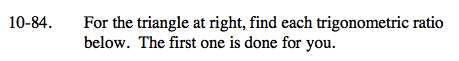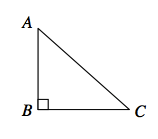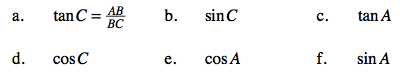### Home > GC > Chapter 10 > Lesson 10.2.3 > Problem10-84A

10-84A.Refer to the Math Notes box in Lesson 5.1.2 if you need help remembering any trigonometric ratios.${\text{cos}C = \frac{BC}{AC}}$

${\text{sin}A = \frac{BC}{AC}}$